# Equilibrium of bodies connected by cord and pulley – application of Newton’s first law problems and solutions

1. A box of mass 5 kg is on an inclined plane at an angle 30o. The box supported by a cord. Determine the tension force (T) and the normal force (N)!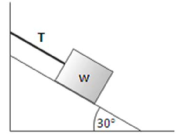Solution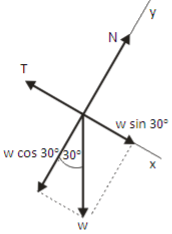Fx = 0

T – w sin 30o = 0

T = w sin 30o

T = (5 kg)(9.8 m/s2) sin 30o

T = (49)(0.5)

T = 24.5 Newton

Fy = 0

N – w cos 30o = 0

N = w cos 30o

N = (49)(0.87)

N = 43 Newton

[irp]

2. Two objects of mass m1 = m2 = 2 kg, connected by a massless string over a frictionless pulley. Find the tension force T1 and T2.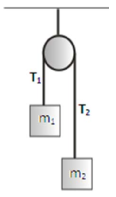Solution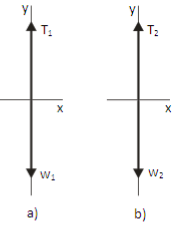(a) Free-body diagram for object 1 (b) Free-body diagram for object 2

Apply Newton’s first law to object 1 :

Fy = 0

T1 – w1 = 0

T1 = w1 = m1 g = (2 kg)(9.8 m/s2) = 19.6 N

Apply Newton’s first law to object 2 :

Fy = 0

T2 – w2 = 0

T2 = w2 = m2 g = (2 kg)(9.8 m/s2) = 19.6 N

T1 = T2 = 19.6 N.

[irp]

3. An object of weight wA = 30 N and an object of weight wB = 40 N, are attached by a lightweight cord that passes over a frictionless pulley of the negligible mass. Determine the coefficient of the maximum static friction between wB and inclined surface, if the system is at rest.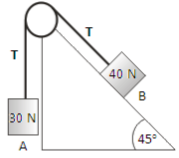Solution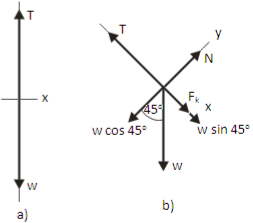(a) Free-body diagram for object wA (b) Free-body diagram for object wB

Apply Newton’s first law to object wA in vertical (y) direction :

Fy = 0 (no acceleration in vertical direction)

T – wA = 0

T = wA = 30 Newton

Apply Newton’s first law to object wB in vertical (y) direction :

Fy = 0

N – wB cos 45o = 0

N = wB cos 45o = (40)(0.7) = 28 Newton

Apply Newton’s first law to object wB in horizontal (x) direction :

Fx = 0

Fk + wB sin 45o – T = 0

μs N + wB sin 45o – T = 0

μs (28) + (40)(0.7) – 30 = 0

μs (28) + 28 – 30 = 0

μs (28) = 30 – 28

μs (28) = 2

μs = 2 / 28

μs = 0.07

The coefficient of the maximum static friction between wB and inclined surface = 0.07.

[wpdm_package id=’490′]